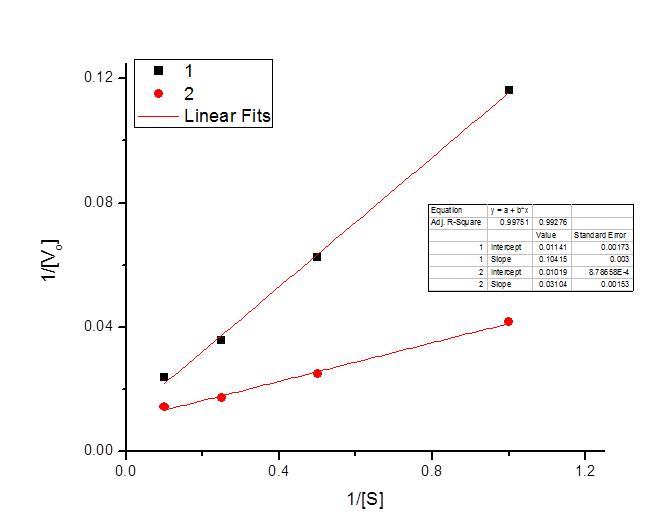# Solutions 9

$$\newcommand{\vecs}{\overset { \rightharpoonup} {\mathbf{#1}} }$$ $$\newcommand{\vecd}{\overset{-\!-\!\rightharpoonup}{\vphantom{a}\smash {#1}}}$$$$\newcommand{\id}{\mathrm{id}}$$ $$\newcommand{\Span}{\mathrm{span}}$$ $$\newcommand{\kernel}{\mathrm{null}\,}$$ $$\newcommand{\range}{\mathrm{range}\,}$$ $$\newcommand{\RealPart}{\mathrm{Re}}$$ $$\newcommand{\ImaginaryPart}{\mathrm{Im}}$$ $$\newcommand{\Argument}{\mathrm{Arg}}$$ $$\newcommand{\norm}{\| #1 \|}$$ $$\newcommand{\inner}{\langle #1, #2 \rangle}$$ $$\newcommand{\Span}{\mathrm{span}}$$ $$\newcommand{\id}{\mathrm{id}}$$ $$\newcommand{\Span}{\mathrm{span}}$$ $$\newcommand{\kernel}{\mathrm{null}\,}$$ $$\newcommand{\range}{\mathrm{range}\,}$$ $$\newcommand{\RealPart}{\mathrm{Re}}$$ $$\newcommand{\ImaginaryPart}{\mathrm{Im}}$$ $$\newcommand{\Argument}{\mathrm{Arg}}$$ $$\newcommand{\norm}{\| #1 \|}$$ $$\newcommand{\inner}{\langle #1, #2 \rangle}$$ $$\newcommand{\Span}{\mathrm{span}}$$$$\newcommand{\AA}{\unicode[.8,0]{x212B}}$$

## Q9.1

Which of the following assumptions are made in Michaelis-Menten kinetics?

1. The concentration of the enzyme is negligible compared to the concentration of the substrate.
2. The substrate is not limiting.
3. The amount of product is small compared to the amount of substrate available.
4. All of the above.
5. None of the above.

## Q9.2

﻿ What is $$V_{max}$$?

1. The concentration of S at which the enzyme becomes saturated.
2. The velocity at which enzyme E is saturated with substrate S.
3. The initial velocity of the enzyme at v=[S].
4. One half of the limiting enzymatic rate.

## Q9.3

What is $$K_m$$?

1. The concentration of substrate at which $$v=[S]$$.
2. The amount of substrate consumed per unit time.
3. The point on the graph at which half the substrate has been consumed.
4. The substrate concentration at which $$v = 1/2 V_{max}$$.

## Q9.4

How would the ten-fold decrease in enzyme concentration affect the observed $$K_m$$?

1. The $$K_m$$ will be unchanged.
2. The $$K_m$$ will increase ten fold.
3. The $$K_m$$ will decrease ten fold.
4. The $$K_m$$ will have to be recalculated from the new data.

## Q9.5

You have isolated the enzyme larsenase from the rare parasite (Facultium ignorantus). This enzyme cleaves the first two numbers off of four digit dates. You flex your skills as an enzymologist and come up with the following graph of initial velocity as a function of substrate concentration:a) From the graph, estimate $$K_m$$.

• 5
• 7
• 17
• 35

b) What is the approximate value of $$V_{max}$$?

• 5
• 7
• 17
• 35

c) Is larsenase an allosteric enzyme? Explain.

• Yes, because allosteric enzymes don't follow Michaelis-Menten kinetics.
• No, because allosteric enzymes don't follow Michaelis-Menten kinetics.
• Yes, because the graph of $$V_o$$ vs. $$[S]$$ is a sigmoidal curve.
• No, because the graph of $$V_o$$ vs. $$[S]$$ is a sigmoidal curve.

## Q9.6

A specific enzyme-catalyzed reaction, $$V_{max} = 0.2 \; mol/sec$$ and $$K_m = 5 \;mM$$. Assume the enzyme shows standard Michaelis-Menten kinetics.

1. What is the rate of the reaction when $$[ S ] = 10\; mM$$ ?\

$V_{max} = .2 mol/s = 200 mmol/s$

$K_m = 5 mM$

$[S] = 10 mM$

$V_0 = \frac{V_{max}*[S]}{K_m + [S]} = 133.333 mmol/s = .133333 mol/s$

b. Draw a Michaelis-Menten plot of the reaction kinetics, labeling the axes and giving values for the point where you can determine $$K_m$$.

## Q9.7

The following data were obtained for an enzyme in the absence of an inhibitor and in the presence of an inhibitor.

 $$[S] \; (mm)$$ $$V_o \; \text{(Experiment one)}$$ $$V_o \; \text{(Experiment two)}$$ 1 8.6 24 2 16 40 4 28 58 10 42 70

Draw a Lineweaver-Burke plot of the reaction kinetics, labeling the axes and giving values for the two points where each line crosses the axes.$x_1 intercept = -\frac{.01019}{.03104} = -.3279$

$x_2 intercept = -\frac{.01141}{.10415} = -.1095$

## Q9.8

What is the chemical basis of enzyme specificity?

The chemical basis of enzyme specificity is the nature and design of the enzyme active site.

Solutions 9 is shared under a CC BY-NC-SA 4.0 license and was authored, remixed, and/or curated by LibreTexts.